×

Search anything:

# Run-time environment: Accessing non-local data on Stack

#### Compiler DesignIn this article, we take a look at how a procedure accesses data used within it but doesn't belong to it.

1. Introduction.
2. Data access without nested procedures.
3. Issues with nested procedures.
4. Nested procedure declarations
5. Nesting depth.
8. Access links for procedure parameters.
9. Displays.

## Introduction.

In this article we shall first take a look at how access of non-local on the stack is done in the C programming language then transition into ML functional programming language since it permits nested functions and function declarations as first-class objects whereby a function can take another function as an argument and return a function as its value.

This is possible by the modifying the implementation of the run-time stack as we shall come to see.

## Data access without nested procedures.

In C languages, variables are defined either within a single function(local variables) or outside the function(global), on the other hand it is impossible to declare a procedure whose scope is within another procedure.

A global variable v has a scope consisting of all functions that follow the declaration of v except where there is a local definition of v's identifier.
Variables declared within a function will have a scope consisting of that function or part of it if the function has a nested block.

Languages which do not allow nested procedure declarations allocate storage variables and accesses them in the following way;

1. Global variables are allocated static storage meaning that the locations of these variables will be fixed and are known at compile time, therefore to access global variables to the executing procedure, we use a statically determined address.
2. Names are local to the activation at the top of the stack and may be accessed through the top_sp(from the prerequisite article) pointer of the stack.

A advantage of static allocation for global variables is that declared procedures are passed as parameters or returned as results without changing the data access strategy. e.g In C we pass a pointer to the function.

In C's static-scoping rule and without nested procedures, any name that is non-local to a procedure is non-local to all procedures regardless of their activation and if a procedure is returned as a result, any non-local name will reference its statically allocated storage location.

The static-scoping rule states that a procedure is permitted to access variables of the procedure whose declarations surround its own declaration.

## Issues with nested procedures.

Accessing non-local data is a complex task for languages which allow nesting of procedure declarations while using the static-scoping rule.

This is because knowing at compile time that a function p's declaration is immediately nested within a function q won't tell us the positions of their activation records at run-time since when either of the procedures are recursive they may have several activation records.

A static decision finds the declaration which applies to a non-local name x in a nested procedure p, it is made by extending the static-scope rule for blocks.
A dynamic decision finds q's activation from p's activation if x is declared in the enclosing procedure q.

The problem is that this requires additional run-time information about activations, we solve this using access links as we shall come to see.

## Nested procedure declarations.

We introduce the ML programming language since it allows nested procedures.

Some properties of ML

• It is a functional language and thus variables once declared and initialized are not changed except in cases e.g an array whose elements are changed by other functions.
• It supports higher order functions, that is, a function can take functions as arguments, construct and return other functions which can in turn take functions as arguments to any level.
• There are no iterations rather recursions. In ML functions call themselves with progressively higher values until a limit is arrived at.
• Lists and labeled tree structures are supported as primitive data types.
• No variable declaration is required rather these are deciphered at compile time otherwise an error is generated.

Variables with unchangeable values are initialized as follows

``````val<name> = <expresion>
``````

functions

``````fun<name> (<arguments>) = <body>
``````

function bodies

``````let <list of definitions> in <statements> end
``````

val and fun statements are frequently used for definitions.
Function definitions can be nested, e.g, the body of a function p can contain a let-statement which includes the definition of another nested function q.
q can have function definitions within its body which leads to deep nesting of functions.

## Nesting depth.

We define nesting depth 1 as the nesting depth for procedures that are not nested in any other procedures, e.g, all C functions have a nesting depth 1.

If a procedure p is defined within another procedure with a nesting depth i we have a nesting depth of i+1 for procedure p.

An example of quickSort algorithm in ML

``````1. fun sort(input_file, output_file) =
let
2.      val a = array(11, 0);
4.          ... a ...;
5.      fun exchange(i, j) =
6.          ... a ...;
7.      fun quiskSort(m, n) =
let
8.              val v = ...;
9.              fun partition(y, z) =
10.                 ... a ... v ... exchange ...
in
11.             ... a ... v ... partition ... quiskSort
end
in
12.     ... a ... read ... quickSort ...
end;
``````
1. At line (1) the sort function reads an array a of nine integers and sorts them.
It is the outermost function and has a nesting of depth 1.
2. At line (2), we have defined an array a of nine integers by declaring array as the first argument stating that we want an array to have 11 elements, the second argument states that all array elements will initially have a value of 0 which is an integer so we don't have to declare a type.
3. Functions at line (3) and (5) have a nesting depth of 2 since they are defined within a function with a nesting depth of 1.
These functions can access the array and can change the values of a, keep in mind that in ML, array accesses can violate the functional nature of the language.
4. From lines (7) through (11) we see the working of quickSort algorithm, v , the pivot element is declared at line (8).
The partition function at line(10) has a nesting depth of 3 since it's defined inside a function with a nesting depth of 2.
It accesses both the array a and the pivot element, it also calls the function exchange.
5. Line (11) we see quickSort function accessing variables a, v, partition function and itself recursively.
6. Line (12) states that the outer function sort accesses a and calls read and quickSort procedures.

We can directly implement the normal static-scope rule for nested functions by adding a pointer - access link, to each activation record.

That is, if a procedure p is nested within procedure q in the source code, then the access link in any activation will point to the most recent activation of q.
It is important for the nesting depth q to be one less than the nesting depth of p so that the access links form a chain from the activation record at the top of the stack to sequences of activations located at progressively lower depths.
Along this formed chain are all the activations whose data and procedures are accessible to the currently executing procedure.

Now, supposing a procedure p at the top of the stack is at a nesting depth ${n}_{p}$ and p needs to access x - an element defined within a procedure q surrounding p and has the nesting depth ${n}_{p}$, We start the activation record for p at the top of the stack and follow the access link ${n}_{p}$ - ${n}_{q}$ times from the activation record for q.
This will always be the highest(most recent) activation record for q that currently appears on the stack and it will contain x.
The compiler will know the layout of activation records and thus x will be found at some fixed offset from the position of q's activation record.

Access links for finding non-local data for the quickSort algorithm
We have represented function names using their first letters and shown some of the data that might appear in various ARs as well as access links for each activation.

1. The image below shows the state after sort function calls read() which loads input into the array and quickSort(1, 9) to sort the array.
Access link from quickSort(1,9) will point to the activation record for sort since sort is more closely nested to the function surrounding quickSort().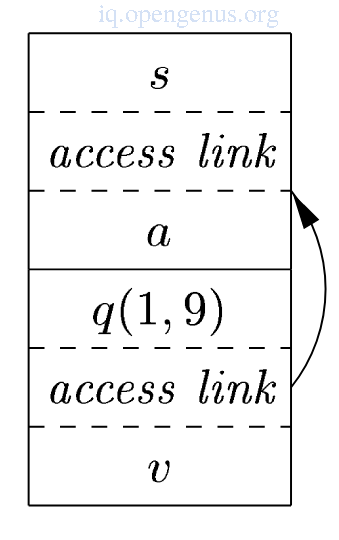1. The recursive calls to quickSort(1, 3) are followed by a call to partition which in turn calls exchange.
quickSort(1, 3)'s access link points to sort just like the access link for quickSort(1, 9).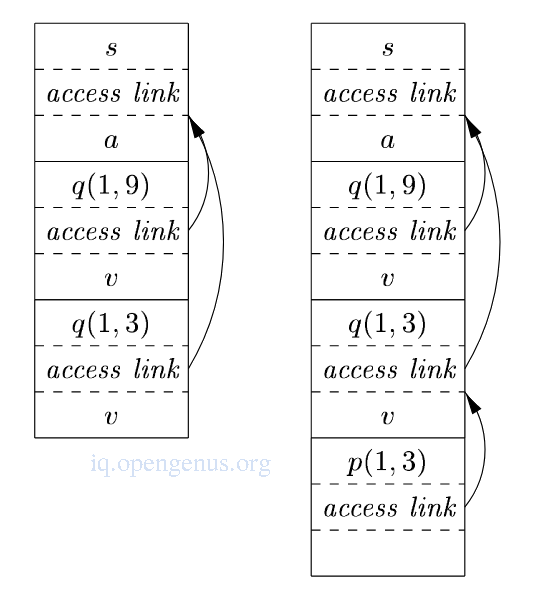1. The access link for exchange bypasses the activation records for quickSort and partition since exchange is nested immediately in sort.
This arrangement is allowed since exchange need access to the array a and the two elements(i, j) it must swap.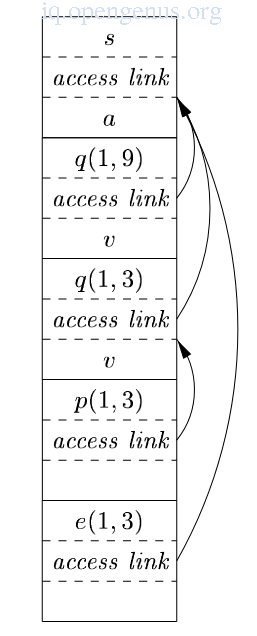When a procedure q calls a procedure p explicitly, two cases exists;

1. The procedure p is at a higher nesting depth than q, Then p must be defined within q or the call by q would not be at a position that is within the scope of the procedure name p and therefore nesting depth of p is exactly one greater than that of q and access link from p must lead to q.

e.g from the quickSort() example, the call of quickSort() by sort() to set up the access links from 1 and the call to partition by quickSort() to create 2(right image).

1. The nesting depth ${n}_{p}$ of p is less than or equal to the nesting depth of ${n}_{q}$ of q.
In the order for the call within q to be in the scope of name p, procedure q must be nested within some procedure r while p is a procedure defined immediately within r.
The top activation record for r is therefore found by following the chain of access links starting from the activation record for q for ${n}_{q}$ - ${n}_{p}$ + 1 hops.
The access link for p must go to this activation of r.
Recursive calls will be included where p = q, in this case the chain of access links is followed for one hop while the access links for p and q remain the same.
e.g Call for quickSort(1,) by quickSort(1, 9) sets up 2(Right-hand side)
This also includes the case for mutually recursive calls whereby two or more procedures are defined within a common parent.

An equivalent way for discovering access links is to follow access links for ${n}_{q}$ - ${n}_{p}$ hops and copy the access link found in the record.

## Access links for procedure parameters.

When a procedure p is passed to another procedure q as a parameter and q calls its parameter(calls p in the activation of q), it is possible that q does not know the context in which p appears in the program and therefore impossible for q to know how to set the access link for p.

We solve this problem by the following, when procedures are used as parameters, the caller needs to pass the name of the procedure-parameter along with the proper access links for the parameter.

The caller will always know the link because if p is passed by procedure r as an actual parameter, p must then be named accessible to r and therefore r can determine the access link for p as if p were being directly called by r

We have the function a which has nested functions b and c
Function b calls a function-valued parameter
Function c defined a function d and hen call b with the parameter d

``````fun a(x) =
let
fun b(f) =
... f ... ;
fun c(y) =
let
fun d(z) = ...
in
... b(d) ...
end
in
... c(1) ...
end;
``````

Actual parameters carrying access links with them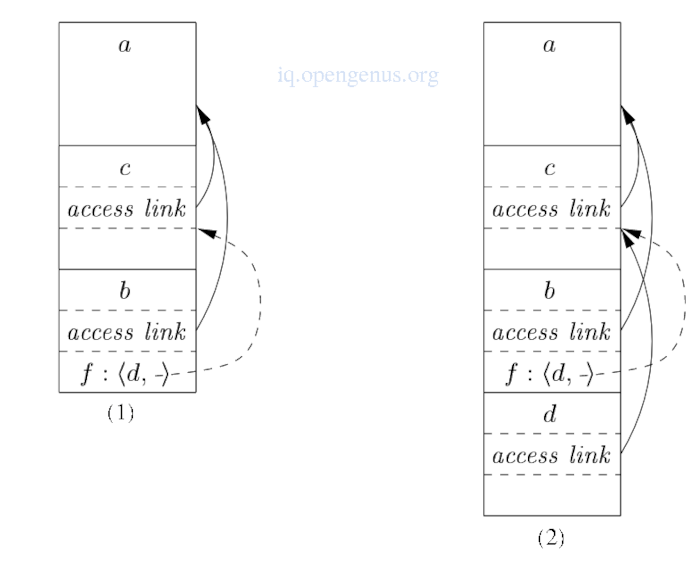## Displays.

access-link approach to non-local data have a drawback that is the nesting depth gets large, we follow long chains of links to reach the needed data.
To improve on this, we can use an auxilliary array d which we call a display and will contain a pointer for each nesting depth.

We expect that at all times d[i] is a pointer to the highest activation record on the stack for any procedure at nesting depth i.

Maintaining the display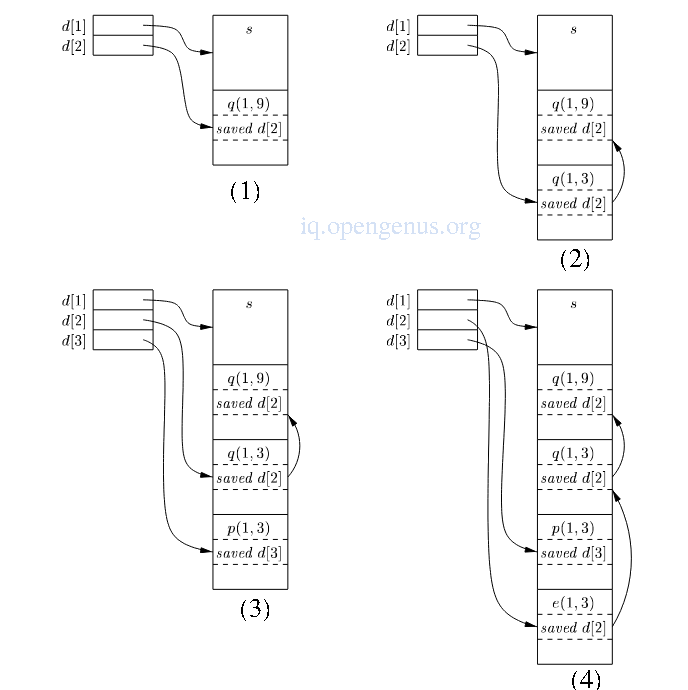manipulating the display

1. sort at depth 1 calls quickSort(1, 9) at depth 2,
The activation record for quicksort will have a place to store the old value of d indicated as saved d, in this case this pointer is null since there was no prior activation record at depth 2.

2. quickSort(1,9) calls quickSort(1,3) and since the ARs for both calls are at depth2, we store the pointer to quickSot(1, 9) previously in d in the record for quickSort(1, 3), d now points to quickSort(1, 3).

3. partition at depth 3 is called again, we use slot d in the display and point it to the AR for partition.
The record for partition has a slot for a former value of d but there is none in this case therefore the pointer remains null.

4. partition calls exchange at depth 2 therefore its AR stores the old pointer d which goes to the activation record for quickSort(1, 3)

From (4) We see the display d with d holding a pointer to the activation record for sort, highest and only activation record for a nesting depth of 1.
d holds a pointer to the activation record for exchange the highest record for functions with nested depth of 2.
d points to partition, the highest record at depth 3.

An advantage of this is that if procedure p is executing and needs to access element x belonging to a procedure q, we look only at d[i] where i is the nesting depth of q and follow the pointer d[i] to the activation record for q where x will be found at a known offset.
The compiler will know i and therefore can generate code to access x using d[i] and x's offset from the top of the AR for q and therefore the code doesn't need to follow a long chain of access links.

We save previous values of display entries in new AR so as to maintain the display correctly.
If procedure p at depth ${n}_{p}$ is called and its activation record is not the first on the stack for a procedure at that depth, the activation record for p will be required to hold previous values of d[${n}_{p}$], while d[${n}_{p}$] itself is set to point to the activation of p.
When p returns, its AR is removed from the stack and we restore d[${n}_{p}$] to have its value prior to the call.

## Summary.

Accessing non-local data is compilicated in programming languages where a procedure is declared inside another procedure.